Throughout,will denote a bounded domain inorIn factwill be assumed to be a polygonor polyhedronWe will be concerned with the problem of finding an eigenvalueand eigenfunctionsatisfyingfor all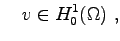(1.1) whereand(1.2) Here, the matrix-valued functionis required to be uniformly positive definite, i.e.for allwithand all(1.3) The methods which we describe below can be extended to piecewise smooth coefficientsis piecewise constant onand that the jumps inare aligned with the meshes(introduced below), for allThe scalar functionis required to be bounded above and below by positive constants for all, i.e.for all(1.4) Throughout the paper, for any polygonal (polyhedral) subdomain ofand any,andwill denote the standard norm and seminorm in the Sobolev space. Alsodenotes theinner product. We also define the energy norm induced by the bilinear form: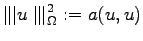for allwhich, by (1.3), is equivalent to theseminorm. (The equivalence constant depends on the contrast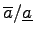, but we are not concerned with this dependence in the present paper.) We also introduce theweighted norm:and note the norm equivalence(1.5) Rewriting the eigenvalue problem(1.1) in standard normalised form, we seeksuch that(1.6) By the continuity ofandand the coercivity ofonit is a standard result that (1.6) has a countable sequence of non-decreasing positive eigenvalues,with corresponding eigenfunctionsWe will need some additional regularity for the eigenfunctions, which will be achieved by making the following regularity assumption for the elliptic problem induced by: Assumption 1.1 We assume that there exists a constantandwith the following property. For, ifsolves the problemfor all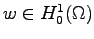, then. Assumption 1.1 is satisfied withwhenis constant (or smooth) andis convex. In a range of other practical cases, for examplenon-convex, orhaving a discoutinuity across an interior interface. Under Assumption 1.1 it follows that the eigenfunctionsof the problem (1.6) satisfyTo approximate problem(1.6) we use the continuous linear finite element method. Accordingly, let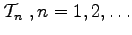denote a family of conforming triangular () or tetrahedral () meshes on. Each mesh consists of elements denoted. We assume that for each,is a refinement of. For a typical elementof any mesh, its diameter is denotedand the diameter of its largest inscribed ball is denoted. For each, letdenote the piecewise constant mesh function on, whose value on each elementisand let. Throughout we will assume that the family of meshesis shape regular, i.e. there exists a constantsuch thatfor alland all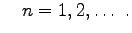(1.7) In the later sections of the paper thewill be produced by an adaptive process which ensures shape regularity. We letdenote the usual finite dimensional subspace of, consisting of all continuous piecewise linear functions with respect to the mesh. Then the discrete formulation of problem (1.6) is to seek the eigenpairssuch that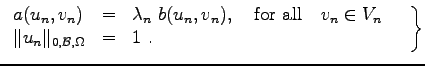(1.8) The problem (1.8) haspositive eigenvalues (counted according to multiplicity) which we denote in non-decreasing order as.